# Newton's method for root-finding for a function of one variable

(Redirected from Newton's method)

## Definition

Newton's method (also known as the Newton-Raphson method) is a root-finding algorithm that can be applied to a differentiable function whose derivative function is known and can be calculated at any point. It is closely related to the secant method, but has the advantage that it requires only a single initial guess.

Note that on this page, we use parenthesized superscripts to denote the iterates of the method, rather than subscripts. This is to keep notation consistent with the multiple-variable case, where subscripts are used for coordinates. Parenthesized superscripts should not be confused with exponents.

## Related methods

Method Change in problem statement Change in method
Newton's method for root-finding for a vector-valued function of a vector variable We have multiple real-valued functions, each of multiple variables. We can model this as a vector-valued function of a vector variable. Division by$f'$ is replaced by left multiplication by the inverse of the Jacobian matrix of the vector-valued function.
Newton's method for optimization of a function of one variable Instead of finding where the function takes the value zero, we are interested in determining the minimum value of a function. We apply Newton's method to the derivative of the function, Thus, instead of the iteration$x^{(k+1)} = x^{(k)} - \frac{f(x^{(k)})}{f'(x^{(k)})}$, we have$x^{(k+1)} = x^{(k)} - \frac{f'(x^{(k)})}{f''(x^{(k)})}$.
Newton's method for optimization of a function of multiple variables Optimization, but now for a single real-valued function of multiple variables. We apply Newton's method for root-finding to the gradient vector of the function. The step is therefore obtained by multiplying the inverse of the Hessian matrix with the gradient vector.

## Iterative step

The initial guess$x^{(0)}$ for the root needs to be supplied to initiate the method.

For any nonnegative integer$k$, define:$x^{(k+1)} := x^{(k)} - \frac{f(x^{(k)})}{f'(x^{(k)})}$

Geometrically, if we consider the tangent line at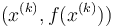$(x^{(k)},f(x^{(k)}))$ to the graph of$f$, then$x^{(k+1)}$ is the$x$-coordinate of the point of intersection of this line with the$x$-axis. In particular, Newton's method would converge in one step if$f$ were a linear function.

Some associated terminology:

• We call the quantity$\left| \frac{f(x^{(k)})}{f'(x^{(k)})} \right|$ the step size. Large step sizes mean big changes to our guesses.
• The step direction is given by the sign of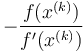$- \frac{f(x^{(k)})}{f'(x^{(k)})}$. The step direction depends on the signs of both$f$ and$f'$.

## Algebraic observations about step size

### Relation with logarithmic derivative and condition number

• The step size$\left| \frac{f(x^{(k)})}{f'(x^{(k)})} \right|$ is the absolute value of the reciprocal of the logarithmic derivative of$f$ at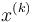$x^{(k)}$.
• The fractional change incurred in each iteration is given by the condition number of$f$ at$x^{(k)}$.

## Termination

We typically choose to terminate based on a criterion of the form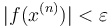$|f(x^{(n)})| < \varepsilon$ for some small enough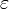$\varepsilon$.

## Failure analysis

The failure analysis could be of the following forms:

• The algorithm throws up an error at some point, i.e., we simply aren't able to compute$x^{(n)}$ for some$n$.
• The algorithm cycles between a set of points and therefore does not make progress.
• The algorithm continues just fine without cycling, but it does not converge to a root.
• The algorithm converges, but very slowly, so that the convergence is not helpful in practice.

### Throwing up an error

The algorithm throws up an error in computing$x^{(k+1)}$ if and only if$x^{(k)}$ is a critical point for the function. There are two subcases:

• The derivative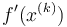$f'(x^{(k)})$ exists and equals zero.
• The derivative$f'(x^{(k)})$ is undefined.

In both cases, the formula for calculating$x^{(k+1)}$ does not work.

(Note that point of local extremum implies critical point, so if we start with, or reach, a point of local extremum for the function, we will throw up an error).

## Sensitivity to the form in which the function is presented

Unlike the case of the bisection method, the qualitative behavior of Newton's method is not invariant under pre-composition or post-composition with monotone transformations. This is because the size of the step moved depends on attributes of the function that are not preserved under arbitrary monotone transformations.

### Invariance under scalar multiplication

The qualitative behavior of Newton's method (and in particular, the sequence of guesses we get by iterating the method with a given starting point) is invariant under scalar multiplication. In other words, the functions$f$ and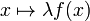$x \mapsto \lambda f(x)$, for a constant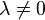$\lambda \ne 0$, behave the same way. This is modulo the caveat about numerical precision. Note, however, that given a particular function, multiplying it by a constant can never improve the precision (because we cannot get more information than already exists) so the key point remains that replacing the function by a scalar multiple doesn't help.

### Effect of power maps

If we replace the function$f$ by a function of the form$g(x) = (f(x))^r$, then this affects Newton's method in the following predictable manner: the step sizes become smaller, even though the set of roots does not change. Therefore, we should expect convergence to be slower in general.

Explicitly, recall that the step size in Newton's method is the reciprocal of the logarithmic derivative. By the chain rule for logarithmic derivatives, post-composing with a power function just multiplies the logarithmic derivative by the condition number for the power function, which is$r$. So, the Newton's method step gets divided by$r$.

This suggests a remedy: if we know that our function has a zero of multiplicity$r$, we deliberately adjust for the small step sizes of Newton's method by multiplying the suggested step size by$r$.

### Covariance under affine transformations of the domain

Consider a function$f$ and a a function$x \mapsto f(mx + c)$ for$m \ne 0$ and$c \in \R$. The functions behave the same way with respect to Newton's method, provided that we adjust the values of$x$ by the same affine transformation. In other words, the sequence of guesses for the second function are obtained by mapping the sequence of guesses for the first function under the mapping$t \mapsto (1/m) (t - c)$.

## Convergence rate

### Convergence for linear functions

For a linear function, Newton's method reaches the exact value of the unique root in a single step, i.e., regardless of the value of$x^{(0)}$, the value of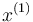$x^{(1)}$ is the desired root.

#### Algebraic explanation

To see this explicitly, consider a linear function: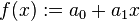$f(x) := a_0 + a_1x$

Then, for any initial value$x^{(0)}$, we have:$f'(x^{(0)}) = a_1$

Thus, we get: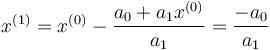$x^{(1)} = x^{(0)} - \frac{a_0 + a_1x^{(0)}}{a_1} = \frac{-a_0}{a_1}$

Also, the unique solution to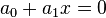$a_0 + a_1x = 0$ is$\frac{-a_0}{a_1}$.

#### Geometric explanation

Geometrically, Newton's method involves constructing the tangent line through the point on the graph corresponding to the current guess for the root, and determining the point of intersection of that line with the$x$-axis.

For a linear function, the tangent line to the graph of the function through any point on it coincides with the graph of the function itself. Therefore, the intersection of the tangent line with the$x$-axis coincides with the intersection of the graph with the$x$-axis, and therefore with the root.

For further information, refer: Newton's method for quadratic functions

Based on the discussion on sensitivity to the form in which the function is presented, there are essentially three types of qualitative behavior:

Case on nature of quadratic function Relation between initial guess$x^{(0)}$ and root of convergence (assume that we do not start at a root) Monotonicity of convergence Order of convergence Numerical rate of convergence for that order
One root with multiplicity two, i.e., the polynomial is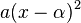$a(x - \alpha)^2$ for some$a,\alpha \in \R$,$a \ne 0$ Converges to the root, but does not reach it in finitely many steps monotonic from the outset 1 1/2
Two distinct real roots, i.e., the polynomial is$a(x - \alpha)(x - \beta)$ for some$a,\alpha,\beta \in \R$,$a \ne 0$,$\alpha < \beta$ Converges to the root it is closer to, but does not reach it in finitely many steps. In particular, converges to$\alpha$ if$x^{(0)} < (\alpha + \beta)/2$, converges to$\alpha$. If$x^{(0)} > (\alpha + \beta)/2$, converges to$\beta$. monotonic after at most one initial step 2 1/2
No real root No root to converge to, chaotic behavior -- -- --

### Some cases where it's particularly easy to reason about convergence, even outside an immediate neighborhood

Case What we can conclude about convergence Further remarks
The part of the domain of a function to the immediate right of a root where the function is increasing and concave up (applies as long as the function stays increasing and concave up). The same holds if "increasing and concave up" is replaced by "decreasing and concave down" Newton's method converges to the root. The sequence of guesses monotonically decreases towards the root. In particular, for a polynomial function that completely factors over the reals into linear factors, this holds for the right-most root: starting with any point to its right, one converges to that root.
The part of the domain of a function to the immediate left of a root where the function is increasing and concave down (applies as long as the function stays that way). The same holds if "increasing and concave down" is replaced by "decreasing and concave up" Newton's method converges to the root. The sequence of guesses monotonically increases towards the root. In particular, for a polynomial function that completely factors over the reals into linear factors, this holds for the left-most root: starting with any point to its left, one converges to that root.
The part of the domain of a function to the immediate left of the right-most root, that has the further property that the function is increasing and concave up on the immediate left (including where one starts) and the entire domain right of the root. (Everything continues to hold if we replace "increasing and concave up" with "decreasing and concave down") Newton's method converges to the root. The first iteration of the method gets us to the right of the root, after which it monotonically decreases to the root. In particular, for a polynomial function that completely factors over the reals into linear factors, the part of the domain to the right of the right-most root of the derivative converges to the right-most root of the function.
The part of the domain of a function to the immediate right of the left-most root, that has the further property that the function is increasing and concave down on the immediate right (including where one starts) and the entire domain left of the root. (Everything continues to hold if we replace "increasing and concave down" with "decreasing and concave up") Newton's method converges to the root. The first iteration of the method gets us to the left of the root, after which it monotonically increases to the root. In particular, for a polynomial function that completely factors over the reals into linear factors, the part of the domain to the left of the left-most root of the derivative converges to the right-most root of the function.